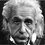Find the minimum value!

$If{\kern 1pt} {x^2}{\kern 1pt} + {\kern 1pt} 2xy{\kern 1pt} - {\kern 1pt} {y^2} = {\kern 1pt} 6.Then{\kern 1pt} {\kern 1pt} find{\kern 1pt} {\kern 1pt} the{\kern 1pt} {\kern 1pt} minimum{\kern 1pt} {\kern 1pt} value{\kern 1pt} {\kern 1pt} of{\kern 1pt} {({x^2} + {y^2})^2}?$ where x and y are real numbers.Note by Kiran Patel
6 years, 3 months ago

This discussion board is a place to discuss our Daily Challenges and the math and science related to those challenges. Explanations are more than just a solution — they should explain the steps and thinking strategies that you used to obtain the solution. Comments should further the discussion of math and science.

When posting on Brilliant:

• Use the emojis to react to an explanation, whether you're congratulating a job well done , or just really confused .
• Ask specific questions about the challenge or the steps in somebody's explanation. Well-posed questions can add a lot to the discussion, but posting "I don't understand!" doesn't help anyone.
• Try to contribute something new to the discussion, whether it is an extension, generalization or other idea related to the challenge.
• Stay on topic — we're all here to learn more about math and science, not to hear about your favorite get-rich-quick scheme or current world events.

MarkdownAppears as
*italics* or _italics_ italics
**bold** or __bold__ bold

- bulleted
- list

• bulleted
• list

1. numbered
2. list

1. numbered
2. list
Note: you must add a full line of space before and after lists for them to show up correctly
paragraph 1

paragraph 2

paragraph 1

paragraph 2

> This is a quote
This is a quote
# I indented these lines
# 4 spaces, and now they show
# up as a code block.

print "hello world"
# I indented these lines
# 4 spaces, and now they show
# up as a code block.

print "hello world"
MathAppears as
Remember to wrap math in $$...$$ or $...$ to ensure proper formatting.
2 \times 3 $2 \times 3$
2^{34} $2^{34}$
a_{i-1} $a_{i-1}$
\frac{2}{3} $\frac{2}{3}$
\sqrt{2} $\sqrt{2}$
\sum_{i=1}^3 $\sum_{i=1}^3$
\sin \theta $\sin \theta$
\boxed{123} $\boxed{123}$

Sort by:

A much easier and convenient way would be this:

$x^2-y^2=6-2xy$

On squaring we have:

$x^4+y^4=36+6(xy)^2-24xy$

Let $N=(x^2+y^2)^2$ for some $N \in \mathbb{R}$ obviously $N \geq 0$

$N=x^4+y^4+2(xy)^2$

$N=36+8(xy)^2-24xy$

Substitute the value of $x^4+y^4=36+6(xy)^2-24xy$

$\large N=8((xy)^2-3xy+\frac{9}{2})$

On factorising:

$\large N=8((xy-\frac{3}{2})^2 -\frac{9}{2} + \frac{9}{4})$

Clearly the minimum occurs at $xy=\frac{3}{2}$

$\Rightarrow N=18$ at $xy=\frac{3}{2}$ where $(x, y)=(\large \sqrt {\frac {\sqrt{18}+3}{2}},\sqrt {\frac {\sqrt{18}-3}{2}})$

We get the minimum to be $18$.

- 6 years, 3 months ago

As with all inequality questions, you need to verify that equality can actually hold. Simply stating that $xy = \frac{3}{2}$ is not sufficient to guarantee that real values of $x$ and $y$ exist. E.g. you could have complex solutions to the equation.

Staff - 6 years, 3 months ago

Yes I was about to do that in the edit.

- 6 years, 3 months ago

Can't it be solved using trigonometry?

- 6 years, 3 months ago

I think $N\geq0$ is incorrect.It must be N>0.

- 6 years, 3 months ago

Yes, if you take into consideration the first equation $N>0$ is more accurate. I stated that $N \geq 0$ without considering the first equation.

- 6 years, 3 months ago

Differentiate the first equation with respect to $x$.

We get: Let $N=(x^2+y^2)^2$

$\large 2x+2y+2x(\frac{dy}{dx})-2y(\frac{dy}{dx})=0$

$\large \frac{dy}{dx}=\frac{x+y}{y-x}$

Similarly differentiating the second equation with respect to $x$:

$\large \frac{dN}{dx}=2(x^2+y^2)(2x+2y\frac{dy}{dx})$

In order to minimize $N$ we have $\frac{dN}{dx}=0$

As such we have:

$x^2+y^2=0$ or $(2x+2y\frac{dy}{dx})=0$

Note that first of the above equation gives us $x=\sqrt{-y^2}$ which is not possible since $x,y \in \mathbb{R}$ .

So we have that:

$\large 2x+2y\frac{dy}{dx}=0$

Substituting for $\frac{dy}{dx}$, gives us:

$y^2-x^2+2xy=0$

Now, $y^2=2xy+x^2-6$

$\Rightarrow 2xy+x^2-6-x^2+2xy=0$

$xy=\frac{3}{2}$

This can be verified to be the minimum value for $xy$ by checking the sign of $\frac{d^2y}{dx^2}$ or maybe we could just use the fact that since it is obvious that $N$ will never reach a maximum so $xy=\frac{3}{2}$ will give the minimum.(I am not exactly sure)

We can re-write $\large N=(x^2+x^2+2xy-6)^2$

$N=4(x^2+xy-3)^2$

Since we have $xy=\frac {3}{2}$

$x^2-y^2=6-3$

Squaring both sides gives us:

$x^4+y^4-2(xy)^2=9$

$x^4+y^4=\frac{27}{2}$

Now,

$N=(x^4+y^4+2(xy)^2$

$\Rightarrow N=\frac{27}{2}+2\frac{9}{4}$

$N=18$ is the minimum value of the expression.

- 6 years, 3 months ago